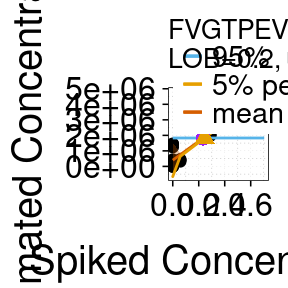# LOB/LOD Estimation Workflow

#### 2021-10-26

library(MSstatsLOBD)
library(dplyr)

This Vignette provides an example workflow for how to use the package MSstatsLOBD.

## Installation

To install this package, start R (version “4.0”) and enter:

if (!requireNamespace("BiocManager", quietly = TRUE))
install.packages("BiocManager")

BiocManager::install("MSstatsLOBD")

# 1 Example dataset

## 1 Introduction

We will estimate the LOB/LOD for a few peptides an assay available on the CPTAC (Clinical Proteomic Tumor Analysis Consortium) assay portal c.f. [@Thomas]. The dataset contains spike in data for 43 distinct peptides. For each peptide, 8 distinct concentration spikes for 3 different replicates are measured. The Skyline files for the assay along with details about the experiment can be obtained from this webpage: https://assays.cancer.gov. The particular dataset examined here (called JHU_DChan_HZhang_ZZhang) can be found at https://panoramaweb.org/labkey/project/CPTAC%20Assay%20Portal/JHU_DChan_HZhang_ZZhang/Serum_QExactive_GlycopeptideEnrichedPRM/begin.view?. It should be downloaded from the MSStats website http://msstats.org/?smd_process_download=1&download_id=548.

The data is then exported in a csv file ($${\tt calibration\_data\_raw.csv}$$) from Skyline. This is done in Skyline by selecting File $$\rightarrow$$ Export $$\rightarrow$$ Report $$\rightarrow$$ QuaSAR input and then clicking Export. The csv file contains the measured peak area for each fragment of each light and heavy version of each peptide. Depending on the format of the Skyline file and depending on whether standards were used, the particular outputs obtained in the csv file may vary. In this particular case the following variables are obtained in the output file $${\tt calibration\_data\_raw.csv}$$: $${\tt File\ Name, Sample\ Name, Replicate\ Name, Protein\ Name, Peptide\ Sequence, Peptide\ Modified\ Sequence,}$$
$${\tt Precursor\ Charge, Product\ Charge,}$$ $${\tt Fragment\ Ion, Average\ Measured\ Retention\ Time}$$, $${\tt SampleGroup, IS\ Spike,}$$ $${\tt Concentration, Replicate,light\ Area, heavy\ Area}$$. A number of variables are byproducts of the acquisition process and will not be considered for the following, i.e.  $${\tt File\ Name, Sample\ Name, Replicate\ Name, SampleGroup, IS\ Spike}$$.
Variables that are important for the assay characterization are detailed below (others are assumed to be self explanatory):

• $${\tt Pepdide sequence}$$ Name of the peptide sequence
• $${\tt Concentration}$$ Value of the known spiked concentration in pmol.
• $${\tt Replicate}$$ Number of the technical replicate
• $${\tt light\ Area}$$ Peak area of the light (measured)
• $${\tt heavy\ Area}$$ Peak area of the heavy (reference) peptide

### 2.1 Load the raw data file and check its content.

head(raw_data)
##       File.Name Sample.Name Replicate.Name          Protein.Name
## 1 Blank_0_1.raw          NA      Blank_0_1 sp|Q9HDC9|APMAP_HUMAN
## 2 Blank_0_2.raw          NA      Blank_0_2 sp|Q9HDC9|APMAP_HUMAN
## 3 Blank_0_3.raw          NA      Blank_0_3 sp|Q9HDC9|APMAP_HUMAN
## 4       A_1.raw          NA            A_1 sp|Q9HDC9|APMAP_HUMAN
## 5       B_1.raw          NA            B_1 sp|Q9HDC9|APMAP_HUMAN
## 6       C_1.raw          NA            C_1 sp|Q9HDC9|APMAP_HUMAN
##   Peptide.Sequence Peptide.Modified.Sequence Precursor.Charge Product.Charge
##   Fragment.Ion Average.Measured.Retention.Time SampleGroup IS.Spike
## 1          y10                           35.19          Bl       NA
## 2          y10                           35.19          Bl       NA
## 3          y10                           35.19          Bl       NA
## 4          y10                           35.19           A       NA
## 5          y10                           35.19           B       NA
## 6          y10                           35.19           C       NA
##   Concentration Replicate light.Area heavy.Area
## 1        0.0000         1      59322          0
## 2        0.0000         2      75627          0
## 3        0.0000         3      62117          0
## 4        0.0576         1      75369          0
## 5        0.2880         1      77955      21216
## 6        1.4400         1      81893     329055

### 2.2 Normalize Dataset

We normalize the intensity of the light peptides using that of the heavy peptides. This corrects any systematic errors that can occur during a run or across replicates. The calculation is greatly simplified by the use of the $${\tt tidyr}$$ and $${\tt dplyr}$$ packages. The area from all the different peptide fragments is first summed then log transformed. The median intensity of the reference heavy peptides $${\tt medianlog2heavy}$$ is calculated. Their intensities should ideally remain constant across runs since the spiked concentration of the heavy peptide is constant. The difference between the median for all the heavy peptide spikes is calculated. It is then used to correct (i.e. to normalize) the intensity of the light peptides $${\tt log2light}$$ to obtain the adjusted intensity $${\tt log2light\_norm}$$. The intensity is finally converted back to original space.

#Select variable that are need
df <- raw_data %>% select(Peptide.Sequence,Precursor.Charge,
Product.Charge, Fragment.Ion,Concentration,
Replicate, light.Area, heavy.Area,
SampleGroup, File.Name)

#Convert factors to numeric and remove NA values:
df <- df %>% mutate(heavy.Area = as.numeric(heavy.Area)) %>%
filter(!is.na(heavy.Area))

#Sum area over all fragments
df2 <- df %>% group_by(Peptide.Sequence, Replicate, SampleGroup,
Concentration, File.Name) %>%
summarize(A_light = sum(light.Area), A_heavy = sum(heavy.Area))
## summarise() has grouped output by 'Peptide.Sequence', 'Replicate', 'SampleGroup', 'Concentration'. You can override using the .groups argument.
#Convert to log scale
df2 <- df2 %>% mutate(log2light = log2(A_light), log2heavy = log2(A_heavy))

#Calculate median of heavy(reference) for a run
df3 <- df2 %>% group_by(Peptide.Sequence) %>%
summarize(medianlog2light = median(log2light),
medianlog2heavy= median(log2heavy))

#Modify light intensity so that the intensity of the heavy is constant (=median) across a run.
df4 <- left_join(df2,df3, by = "Peptide.Sequence") %>%
mutate(log2light_delta = medianlog2light - log2light) %>%
mutate(log2heavy_norm = log2heavy + log2light_delta,
log2light_norm = log2light + log2light_delta) %>%
mutate(A_heavy_norm = 2**log2heavy_norm, A_light_norm = 2**log2light_norm)

#Format the data for MSstats:
#Select the heavy area, concentration, peptide name and Replicate
df_out <- df4 %>% ungroup() %>%
select(A_heavy_norm, Concentration, Peptide.Sequence, Replicate)

#Change the names according to MSStats requirement:
df_out <- df_out %>% rename(INTENSITY = A_heavy_norm,
CONCENTRATION = Concentration,
NAME = Peptide.Sequence, REPLICATE = Replicate)

# We choose NAME as the peptide sequence
head(df_out)
## # A tibble: 6 × 4
##   INTENSITY CONCENTRATION NAME               REPLICATE
##       <dbl>         <dbl> <chr>                  <int>
## 1    34177.        0.0576 AAPAPQEATATFNSTADR         1
## 2   448636.        0.288  AAPAPQEATATFNSTADR         1
## 3        0         0      AAPAPQEATATFNSTADR         1
## 4        0         0      AAPAPQEATATFNSTADR         1
## 5    62968.        0      AAPAPQEATATFNSTADR         1
## 6    43668.        0      AAPAPQEATATFNSTADR         1

# 3 LOB/LOD definitions

## 3.1 Assay characterization procedure

In the following we estimate the LOB and LOD for individual peptides. The first step in the estimation is to fit a function to all the (Spiked Concentration, Measured Intensity) points. When the $${\tt nonlinear\_quantlim}$$ function is used, the function that is fit automatically adapts to the data. For instance, when the data is linear, a straight line is used, while when a threshold (i.e.  a leveling off of the measured intensity at low concentrations) an elbow like function is fit. The fit is called $${\tt MEAN}$$ in the output of function $${\tt nonlinear\_quantlim}$$ as shown in Fig.1. Each value of $${\tt MEAN}$$ is given for a particular $${\tt CONCENTRATION}$$ value $${\tt CONCENTRATION}$$ is thus a discretization of x–Spiked Concentration axis. The lower and upper bound of the 90% prediction interval of the fit are called $${\tt LOW}$$ and $${\tt UP}$$ in the output of $${\tt nonlinear\_quantlim}$$. They correspond respectively to the 5% and 95% percentile of predictions.

The second step in the procedure is to estimate the upper bound of the noise in the blank sample (blue dashed line in Fig. 1). It is found by assuming that blank sample measurements are normally distributed.

## 3.2 LOB/LOD definitions

We define the LOB as the highest apparent concentration of a peptide expected when replicates of a blank sample containing no peptides are measured. This amounts to finding the concentration at the intersection of the fit (which represents the averaged measured intensity) with the 95% upper prediction bound of the noise.

The LOD is defined as the measured concentration value for which the probability of falsely claiming the absence of a peptide in the sample is 0.05, given a probability 0.05 of falsely claiming its presence. Estimating the LOD thus amounts to finding the concentration at the intersection between the 5% percentile line of the prediction interval of the fit (i.e. the lower bound of the 90% prediction interval) and the 95% percentile line of the blank sample. At the LOB concentration, there is an 0.05 probability of false positive and a 50% chance of false negative. At the LOD concentration there is 0.05 probability of false negative and a false positive probability of 0.05 in accordance with its definition. By default, a probability of 0.05 for the LOB/LOD estimation is used but it can be changed, as detailed in the manual.

# 4 Estimation of the LOB/LOD for dataset

## 4.1 LOB/LOD estimation for a non-linear peptide

#Select peptide of interest:  LPPGLLANFTLLR
spikeindata <- df_out %>% filter(NAME == "LPPGLLANFTLLR")

#This contains the measured intensity for the peptide of interest
head(spikeindata)
## # A tibble: 6 × 4
##   INTENSITY CONCENTRATION NAME          REPLICATE
##       <dbl>         <dbl> <chr>             <int>
## 1    26291.        0.0576 LPPGLLANFTLLR         1
## 2   244841.        0.288  LPPGLLANFTLLR         1
## 3        0         0      LPPGLLANFTLLR         1
## 4   774274.        0      LPPGLLANFTLLR         1
## 5   482008.        0      LPPGLLANFTLLR         1
## 6   780792.        0      LPPGLLANFTLLR         1
#Call MSStatsLOD function:
quant_out <- nonlinear_quantlim(spikeindata)

head(quant_out)
##   CONCENTRATION      MEAN       LOW        UP      LOB      LOD   SLOPE
## 1  0.000000e+00  369840.1 -164438.9  931586.0 1.120106 1.262368 2381531
## 2  1.776357e-15  369840.1 -147577.2  916435.0 1.120106 1.262368 2381531
## 3  5.760000e-02  369840.1  338736.3  397424.3 1.120106 1.262368 2381531
## 4  2.880000e-01  369840.1  321218.1  416938.4 1.120106 1.262368 2381531
## 5  7.040283e-01  369840.1  239124.8  505914.0 1.120106 1.262368 2381531
## 6  1.440000e+00 1387627.4 1169887.1 1620774.5 1.120106 1.262368 2381531
##   INTERCEPT          NAME    METHOD
## 1  -5750519 LPPGLLANFTLLR NONLINEAR
## 2  -5750519 LPPGLLANFTLLR NONLINEAR
## 3  -5750519 LPPGLLANFTLLR NONLINEAR
## 4  -5750519 LPPGLLANFTLLR NONLINEAR
## 5  -5750519 LPPGLLANFTLLR NONLINEAR
## 6  -5750519 LPPGLLANFTLLR NONLINEAR

After estimating LOB/LOD we can plot the results.

#plot results in the directory
plot_quantlim(spikeindata = spikeindata, quantlim_out = quant_out, address =  FALSE)
## []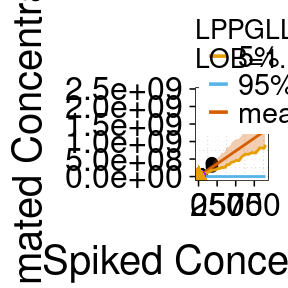##
## []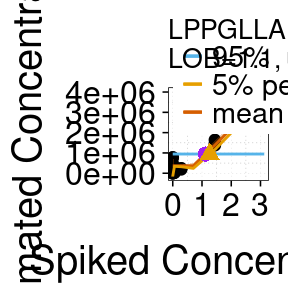The threshold is captured by the fit at low concentrations. The $${\tt MEAN}$$ of the output of the function is the red line (mean prediction) in the plots. $${\tt LOW}$$ is the orange line (5% percentile of predictions) while $${\tt UP}$$ is the upper boundary of the red shaded area. The LOB is the concentration at the intersection of the fit and the estimate for the 95% upper bound of the noise (blue line). A more accurate “smoother” fit can be obtained by increasing the number of points $${\tt Npoints}$$ used to discretize the concentration axis (see manual for $${\tt nonlinear\_quantlim}$$).

The nonlinear MSStats function ($${\tt nonlinear\_quantlim}$$) works for all peptides (those with a linear response and those with a non-linear response). We now examine a peptide with a linear behavior.

## 4.2 LOB/LOD estimation for a linear peptide

#Select peptide of interest:  FLNDTMAVYEAK
spikeindata2 <- df_out %>% filter(NAME == "FVGTPEVNQTTLYQR")

#This contains the measured intensity for the peptide of interest
head(spikeindata2)
FALSE # A tibble: 6 × 4
FALSE   INTENSITY CONCENTRATION NAME            REPLICATE
FALSE       <dbl>         <dbl> <chr>               <int>
FALSE 1   323763.        0.0576 FVGTPEVNQTTLYQR         1
FALSE 2  2036098.        0.288  FVGTPEVNQTTLYQR         1
FALSE 3      205.        0      FVGTPEVNQTTLYQR         1
FALSE 4  1431235.        0      FVGTPEVNQTTLYQR         1
FALSE 5  1244348.        0      FVGTPEVNQTTLYQR         1
FALSE 6  1455085.        0      FVGTPEVNQTTLYQR         1
#Call MSStats function:
quant_out2 <- nonlinear_quantlim(spikeindata2)

head(quant_out2)
##   CONCENTRATION      MEAN       LOW        UP       LOB       LOD   SLOPE
## 1  0.000000e+00  658021.6 -337385.1 1643112.7 0.2626418 0.2720094 5020096
## 2  1.776357e-15  658021.6 -382958.6 1717632.1 0.2626418 0.2720094 5020096
## 3  5.760000e-02  658021.6  544403.0  774783.9 0.2626418 0.2720094 5020096
## 4  2.880000e-01 1973785.0 1924772.4 2021361.2 0.2626418 0.2720094 5020096
## 5  7.040283e-01 4349630.6 4231269.7 4484906.8 0.2626418 0.2720094 5020096
## 6  1.440000e+00 8552602.5 8340749.1 8729696.6 0.2626418 0.2720094 5020096
##   INTERCEPT            NAME    METHOD
## 1  10349525 FVGTPEVNQTTLYQR NONLINEAR
## 2  10349525 FVGTPEVNQTTLYQR NONLINEAR
## 3  10349525 FVGTPEVNQTTLYQR NONLINEAR
## 4  10349525 FVGTPEVNQTTLYQR NONLINEAR
## 5  10349525 FVGTPEVNQTTLYQR NONLINEAR
## 6  10349525 FVGTPEVNQTTLYQR NONLINEAR
#plot results in the directory: "/Users/cyrilg/Desktop/Workflow/Results"
#Change directory appropriately for your computer
plot_quantlim(spikeindata = spikeindata2, quantlim_out  = quant_out2,
address = FALSE)
## []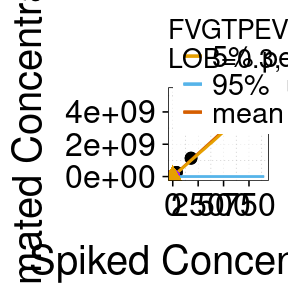##
## []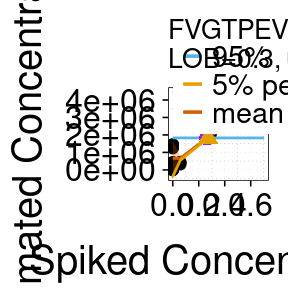The plots indicate that the fit is observed to be linear as the response is linear.

## 4.3 LOB/LOD linear estimation for a non-linear peptide

#Call MSStatsLOD function:
quant_out <- linear_quantlim(spikeindata)

head(quant_out)
##   CONCENTRATION       MEAN        LOW         UP       LOB       LOD   SLOPE
## 1  0.000000e+00 -104154.21 -571738.77  421241.50 0.7599674 0.8613522 2381531
## 2  1.776357e-15 -104154.21 -619378.21  407562.47 0.7599674 0.8613522 2381531
## 3  5.760000e-02  -24617.67  -60207.07    4429.99 0.7599674 0.8613522 2381531
## 4  2.880000e-01  293528.48  249661.86  341050.83 0.7599674 0.8613522 2381531
## 5  7.040283e-01  867998.09  747962.59 1012179.21 0.7599674 0.8613522 2381531
## 6  1.440000e+00 1884259.24 1670844.98 2105815.74 0.7599674 0.8613522 2381531
##   INTERCEPT          NAME METHOD
## 1  -5750520 LPPGLLANFTLLR LINEAR
## 2  -5750520 LPPGLLANFTLLR LINEAR
## 3  -5750520 LPPGLLANFTLLR LINEAR
## 4  -5750520 LPPGLLANFTLLR LINEAR
## 5  -5750520 LPPGLLANFTLLR LINEAR
## 6  -5750520 LPPGLLANFTLLR LINEAR

After estimating LOB/LOD we can plot the results.

#plot results in the directory
plot_quantlim(spikeindata = spikeindata, quantlim_out = quant_out, address =  FALSE)
## []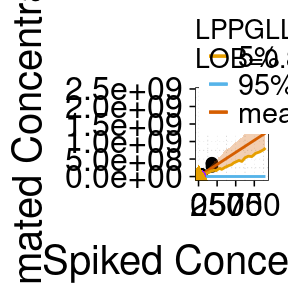##
## []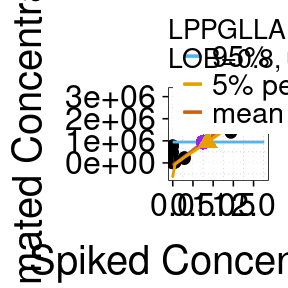## 4.4 LOB/LOD linear estimation for a linear peptide

#Call MSStatsLOD function:
quant_out <- linear_quantlim(spikeindata2)

head(quant_out)
##   CONCENTRATION      MEAN       LOW        UP       LOB       LOD   SLOPE
## 1  0.000000e+00  401694.0 -625507.7 1453061.4 0.2348698 0.2454443 5020096
## 2  1.776357e-15  401694.0 -654701.5 1532274.3 0.2348698 0.2454443 5020096
## 3  5.760000e-02  751722.4  642555.7  853232.3 0.2348698 0.2454443 5020096
## 4  2.880000e-01 2151835.9 2097749.0 2207036.2 0.2348698 0.2454443 5020096
## 5  7.040283e-01 4679990.4 4563853.0 4821391.6 0.2348698 0.2454443 5020096
## 6  1.440000e+00 9152403.3 8940089.8 9355318.4 0.2348698 0.2454443 5020096
##   INTERCEPT            NAME METHOD
## 1  10349527 FVGTPEVNQTTLYQR LINEAR
## 2  10349527 FVGTPEVNQTTLYQR LINEAR
## 3  10349527 FVGTPEVNQTTLYQR LINEAR
## 4  10349527 FVGTPEVNQTTLYQR LINEAR
## 5  10349527 FVGTPEVNQTTLYQR LINEAR
## 6  10349527 FVGTPEVNQTTLYQR LINEAR

After estimating LOB/LOD we can plot the results.

#plot results in the directory
plot_quantlim(spikeindata = spikeindata2, quantlim_out = quant_out, address =  FALSE)
## []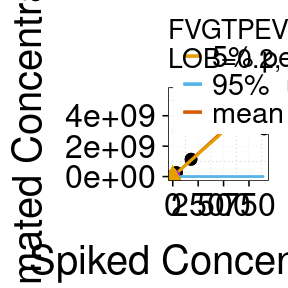##
## []